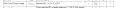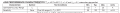# acs712 current sensor

#### mahmut_kelesoglu

Joined Aug 12, 2022
67
ı need to use acs712 current sensor with arduino for sensing the current. as my researches, for calculating the current, first, one have to calculate voltage by using analog output value of the sensor. then, by using this voltage, calculate the current by dividing voltage value to resistance value.
the part that ı want to ask is "ıs it not a cuurent sensor? why it does not give the current value? how can ı calculate the current?"
actuallt my circuit and code runs very well on proteus, but in real circuit, the sensor gives stupid values.
my circuit and code is attached.

#### Attachments

• 12.6 KB Views: 4
• 300 KB Views: 10

#### Ian0

Joined Aug 7, 2020
8,947
Did you take into account that ACS712 is a bi-directional current sensor, and that the output with zero current is half the supply voltage?

#### eetech00

Joined Jun 8, 2013
3,653
ı need to use acs712 current sensor with arduino for sensing the current. as my researches, for calculating the current, first, one have to calculate voltage by using analog output value of the sensor. then, by using this voltage, calculate the current by dividing voltage value to resistance value.
the part that ı want to ask is "ıs it not a cuurent sensor? why it does not give the current value? how can ı calculate the current?"
actuallt my circuit and code runs very well on proteus, but in real circuit, the sensor gives stupid values.
my circuit and code is attached.
The model is not very good.
The real part has a 2.5v offset. The offset has to be removed, usually by an opamp.

#### Ian0

Joined Aug 7, 2020
8,947
The model is not very good.
The real part has a 2.5v offset. The offset has to be removed, usually by an opamp.
If the ACS712 shares the same power supply as the microcontroller then zero current will give an a/d result of 0x8000 (assuming a 16-bit A/D or a left-justfied 16-bit result). All you have to do is subtract 0x8000 from the A/D reading to give a two's complement number.

•ronsimpson

Joined Feb 20, 2016
4,370
A trick you can try is to offset the reading by carefully positioning a magnet near the ACS712 as the device is a Hall sensor. Then you can have the full range for DC.

#### mahmut_kelesoglu

Joined Aug 12, 2022
67
Did you take into account that ACS712 is a bi-directional current sensor, and that the output with zero current is half the supply voltage?
What do you mean? I did not understand.

#### mahmut_kelesoglu

Joined Aug 12, 2022
67
The model is not very good.
The real part has a 2.5v offset. The offset has to be removed, usually by an opamp.
What is this 2.5v ofset?

#### bertus

Joined Apr 5, 2008
22,232

#### mahmut_kelesoglu

Joined Aug 12, 2022
67

#### bertus

Joined Apr 5, 2008
22,232
Hello,

Vout is the voltage that comes out of the ACS sensor.

Bertus

#### mahmut_kelesoglu

Joined Aug 12, 2022
67
Hello,

Vout is the voltage that comes out of the ACS sensor.

Bertus
Out pin voltage of the acs712?

#### bertus

Joined Apr 5, 2008
22,232

#### eetech00

Joined Jun 8, 2013
3,653
What is this 2.5v ofset?
Its is the dc output voltage when the sensed current is zero. So it is added to the output voltage.
The hardware solution is to use an opamp subtractor to remove the offset. Post #4 suggests a software method.

#### eetech00

Joined Jun 8, 2013
3,653
ı need to use acs712 current sensor with arduino for sensing the current. as my researches, for calculating the current, first, one have to calculate voltage by using analog output value of the sensor. then, by using this voltage, calculate the current by dividing voltage value to resistance value.
the part that ı want to ask is "ıs it not a cuurent sensor? why it does not give the current value? how can ı calculate the current?"
actuallt my circuit and code runs very well on proteus, but in real circuit, the sensor gives stupid values.
my circuit and code is attached.

#### mahmut_kelesoglu

Joined Aug 12, 2022
67

#### Attachments

• 120.8 KB Views: 2

#### mahmut_kelesoglu

Joined Aug 12, 2022
67
hi mk,
What do you mean when you say the real circuit will not work.
What are the Vout and Vout2 voltages.?
E
View attachment 278352
ı mean, the sensor write different numbers
for example, first 23.520, then 250.125, then 312.123, then -325.238 .....
ı did not measure Vout and Vout2 values

#### mahmut_kelesoglu

Joined Aug 12, 2022
67
Its is the dc output voltage when the sensed current is zero. So it is added to the output voltage.
The hardware solution is to use an opamp subtractor to remove the offset. Post #4 suggests a software method.
thanks a lot for the answer. ı hope it will help a lot.

#### ronsimpson

Joined Oct 7, 2019
2,796
Use the filter capacitor on pin 6.
It is common to average 10 numbers because of noise.
Do not make hardware answers. Use software.
If you make one circuit you can make it any way that works. But, when we make millions this must be different. We make the "2.5 offset" in EEPROM because some parts need 2.55 and some need 2.45 and you can calibrate so every time, zero current = zero number. (note that "2.5V" is not right, it is actually 1/2 the supply voltage)
Also,
The data sheet has x.x mV/A which depends on which part number you are using. The Data Sheet indicates +/-4% error. We calibrate each part and save the error in EEPROM so all boards read correctly.#### eetech00

Joined Jun 8, 2013
3,653
(note that "2.5V" is not right, it is actually 1/2 the supply voltage)
It is right if VCC=5vBut I agree, I should have stated VCC*0.5 where VCC is the ACS712 supply voltage.